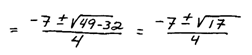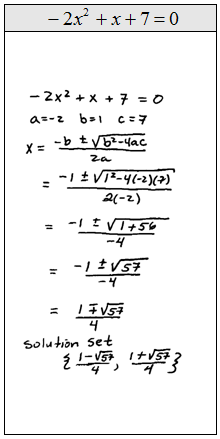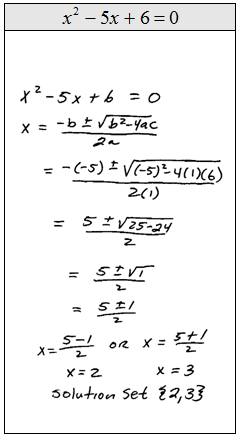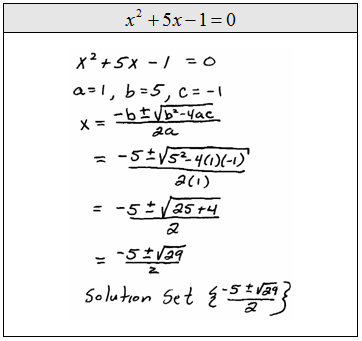## Pages

If a quadratic equation factors then certainly you should solve it by factoring. The problem is that not all quadratic equations factor. When this is the case we could use the quadratic formula to find the solutions. (We will limit our introduction to equations where b^2 - 4ac >= 0 .)Most textbooks provide a nice proof of the quadratic formula. The proof involves solving the general quadratic by completing the square. This study guide will focus on using the formula to solve problems.Step 1: Identify the coefficients a, b, and c.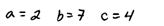Step 2: Write down the formula (you should memorize it).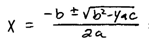Step 3: Substitute in the appropriate values and then evaluate.